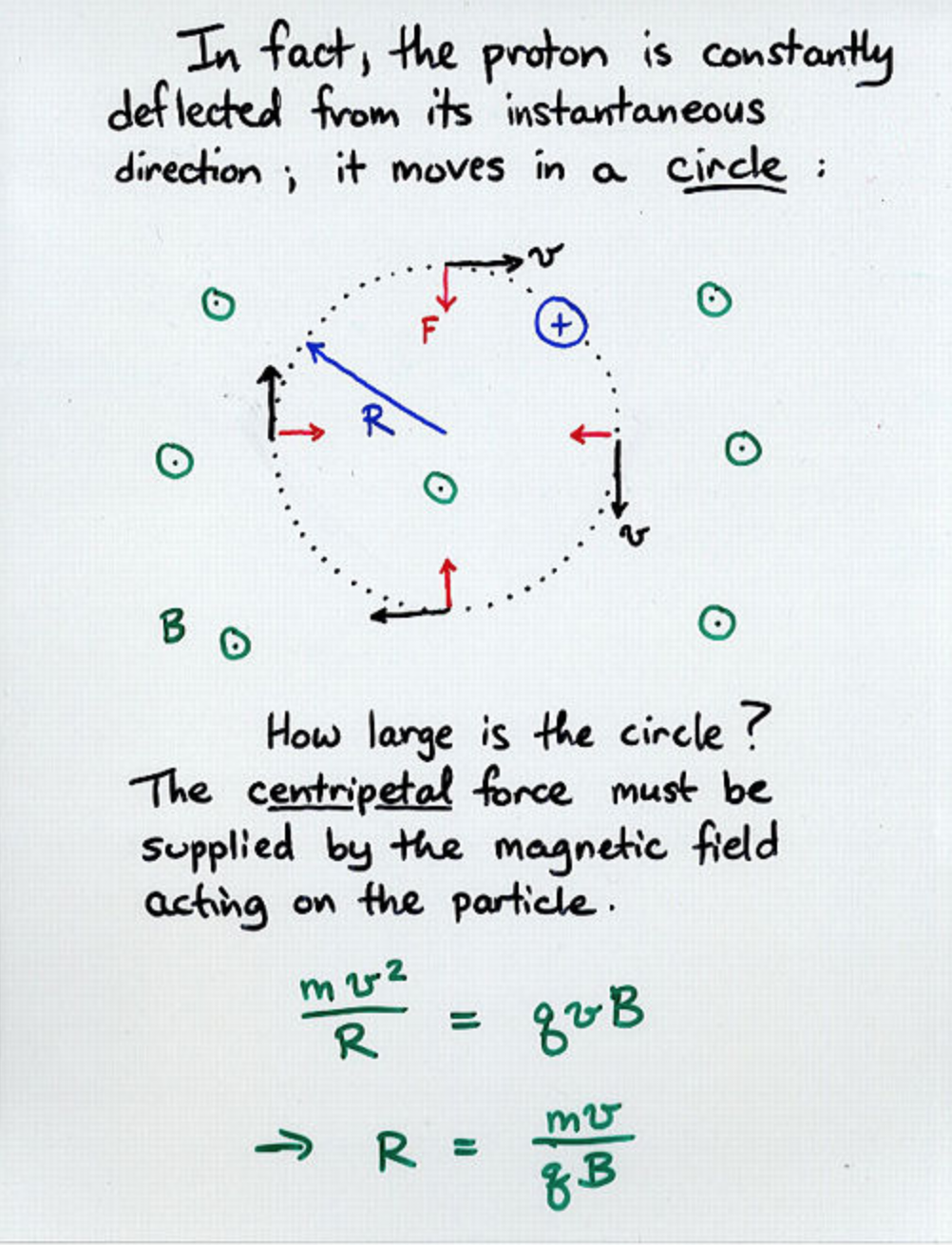Vivekanand Vellanki
0

When a charged particle (electron in this case) enters a uniform magnetic field perpendicular to the field, it ends up moving in a circle with radius:

r=\frac{mv}{cF}where m is the mass of the particle, v is its speed, c is the charge of the particle and F is the magnetic field.

Assuming that the magnetic field is a uniform magnetic, the above should give the answer since the mass and charge of an electron are known.

This is a good picture from the above article:The magnetic force on the electron matches the centripetal force on the electron. This defines the radius of the circle in which the electron moves.